# Braking distance

The car travels at an average speed of 12 km/h and detects an obstacle 10 m in front of it. At 1 m in front of the obstacle it already runs 2 km/h. What is the braking distance? What is the required deceleration for stop in:

A) 1m
B) 1s?

x =  12.96 m
a2 =  0.5556 m/s2

### Step-by-step explanation: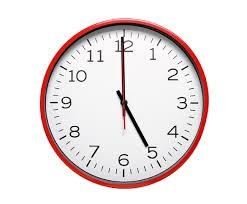Did you find an error or inaccuracy? Feel free to write us. Thank you!Tips to related online calculators
Do you have a linear equation or system of equations and looking for its solution? Or do you have a quadratic equation?
Do you want to convert velocity (speed) units?
Do you want to convert time units like minutes to seconds?

## Related math problems and questions:

• BrakesThe braking efficiency of a passenger car is required to stop at 12.5 m at an initial speed of 40 km/h. What is the acceleration braking by brakes?
• An accelerationThe car goes on a straight road at a speed of 72 km/h. At some point, the driver starts to brake and stops the car in 5 seconds. Find: (a) the acceleration during braking (b) the distance traveled during braking.
• Car crashOn the road, with a maximum permitted speed of 60 km/h, there was a car crash. From the length of the vehicle's braking distance, which was 40 m, the police investigated whether the driver did not exceed that speed. What is the conclusion of the police, a
• Brakes of a carFor the brakes of a passenger car to be effective, it is prescribed that a car be moving on a horizontal road at a speed of 40 km. A car must stop on the track 15.4 m. What is the deceleration of the car?
• Car overtaking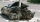A passenger car travels at a speed of 30 m/s, and before it travels freight truck that drives at a constant speed of 10 m/s. Within 30 meters of the truck, the driver of the car finds out that the truck can not overtake. That's why it starts braking with
• DriverThe car driver drives at a speed of 100 km/h, saw the obstacle, and began to brake with a slowing of 5 m/s². What is the length of the pathway to stopping the car when the driver has registered the obstacle with a delay of 0.7 s?
• Deceleration of carThe car has a speed of 60 km/h and after 100 m journey speed of 40 km/h. What is the deceleration of a car if we assume that its movement is constantly slowed down?
• Train speed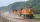The train speed is decreased during 50 sec from 72 km/h to 36 km/h. Assuming that the train movement is equally slowing, find the the acceleration and the distance that it travels at.
• AccelerationThe car accelerates at a rate of 0.5 m/s2. How long travels 400 meters, and what will be its speed?
• Train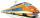The train is running at speeds of 96 km/h. From the beginning of braking to full stop train run for 3.3 minutes. If the train slows the braking equally, calculate the distance from the location where you need to start to brake.
• Car loop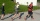The toy car runs at an average speed of 2 m/s. In a minute, it will pass the entire circuit five times. How long is the loop?
• Car driver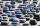The car driver is in town A and is scheduled to be in town B at a specified hour. If it travels at an average speed of 50km/h, it will arrive in city B 30 minutes later. But if he travels at an average speed of 70km/h, he would arrive half an hour earlier
• Traffic collisionWhen investigating a traffic accident, it was found that the driver stopped the vehicle immediately after the accident by constant braking on a 150 m track in 15 seconds. Do you approve that the driver exceeded the permitted speed (50 km/h) in the village
• Average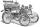What is the average speed of the car, where half of the distance covered passed at speed 66 km/h and the other half at 86 km/h.
• Two citiesThe car goes from city A to city B at an average speed of 70 km/h, back at an average speed of 50 km/h. If it goes to B and back at an average speed of 60 km/h, the whole ride will take 8 minutes less. What is the distance between cities A and B?
• G forcesCalculate deceleration of car (as multiple of gravitational acceleration g = 9.81 m/s2) occurs when a vehicle in a frontal collision slows down uniformly from a speed 111 km/h to 0 km/h in 1.2 meters trajectory.
• Train and car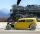The train and the car started at a constant speed to journey. When the train travels 87 km, the car travels 97 km. How many km travels the train when the car travels 87 km?